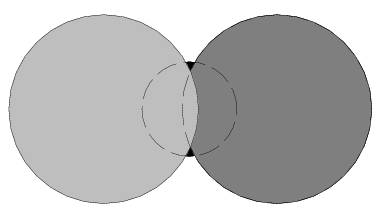## poj1418 Viva Confetti 判断圆是否可见

Viva Confetti
 Time Limit: 1000MS Memory Limit: 10000K Total Submissions: 881 Accepted: 361

Description

Do you know confetti? They are small discs of colored paper, and people throw them around during parties or festivals. Since people throw lots of confetti, they may end up stacked one on another, so there may be hidden ones underneath.

A handful of various sized confetti have been dropped on a table. Given their positions and sizes, can you tell us how many of them you can see?

The following figure represents the disc configuration for the first sample input, where the bottom disc is still visible.Input

The input is composed of a number of configurations of the following form.

x1 y1 r1
x2 y2 r2
...
xn yn rn

The first line in a configuration is the number of discs in the configuration (a positive integer not more than 100), followed by one line descriptions of each disc : coordinates of its center and radius, expressed as real numbers in decimal notation, with up to 12 digits after the decimal point. The imprecision margin is +/- 5 x 10^(-13). That is, it is guaranteed that variations of less than +/- 5 x 10^(-13) on input values do not change which discs are visible. Coordinates of all points contained in discs are between -10 and 10.

Confetti are listed in their stacking order, x1 y1 r1 being the bottom one and xn yn rn the top one. You are observing from the top.

The end of the input is marked by a zero on a single line.

Output

For each configuration you should output the number of visible confetti on a single line.

Sample Input

3
0 0 0.5
-0.9 0 1.00000000001
0.9 0 1.00000000001
5
0 1 0.5
1 1 1.00000000001
0 2 1.00000000001
-1 1 1.00000000001
0 -0.00001 1.00000000001
5
0 1 0.5
1 1 1.00000000001
0 2 1.00000000001
-1 1 1.00000000001
0 0 1.00000000001
2
0 0 1.0000001
0 0 1
2
0 0 1
0.00000001 0 1
0

Sample Output

3
5
4
2
21 /**
2  * code generated by JHelper
4  * @author xyiyy @https://github.com/xyiyy
5  */
6
7 #include <iostream>
8 #include <fstream>
9
10 //#####################
11 //Author:fraud
12 //Blog: http://www.cnblogs.com/fraud/
13 //#####################
15 #include <iostream>
16 #include <sstream>
17 #include <ios>
18 #include <iomanip>
19 #include <functional>
20 #include <algorithm>
21 #include <vector>
22 #include <string>
23 #include <list>
24 #include <queue>
25 #include <deque>
26 #include <stack>
27 #include <set>
28 #include <map>
29 #include <cstdio>
30 #include <cstdlib>
31 #include <cmath>
32 #include <cstring>
33 #include <climits>
34 #include <cctype>
35
36 using namespace std;
37 #define pb(X) push_back(X)
38 #define rep(X, N) for(int X=0;X<N;X++)
39 #define ALL(X) (X).begin(),(X).end()
40
41 //
42 // Created by xyiyy on 2015/8/10.
43 //
44
45 #ifndef JHELPER_EXAMPLE_PROJECT_P_HPP
46 #define JHELPER_EXAMPLE_PROJECT_P_HPP
47
48 const double EPS = 4e-13;
49
50 double add(double a, double b) {
51     if (fabs(a + b) < EPS * (fabs(a) + fabs(b)))return 0;
52     return a + b;
53 }
54
55 class P {
56 public:
57     double x, y;
58
59     P() { }
60
61     P(double x, double y) : x(x), y(y) { }
62
63     P  operator+(const P &p) {
65     }
66
67     P operator-(const P &p) {
69     }
70
71     P operator*(const double &d) {
72         return P(x * d, y * d);
73     }
74
75     P operator/(const double &d) {
76         return P(x / d, y / d);
77     }
78
79     double dot(P p) {
80         return add(x * p.x, y * p.y);
81     }
82
83
84     double abs() {
85         return sqrt(abs2());
86     }
87
88     double abs2() {
89         return dot(*this);
90     }
91
92 };
93
94
95
96 //求两圆的极角 以p为中心
97 double polarangle(P p, P q) {
98     return atan2(q.y - p.y, q.x - p.x);
99 }
100
101
102 #endif //JHELPER_EXAMPLE_PROJECT_P_HPP
103
104 const long double PI2 = 2 * acos(-1.0);
105
106 long double update(double x) {
107     while (x < 0.0)x += PI2;
108     while (x >= PI2)x -= PI2;
109     return x;
110 }
111
112 class poj1418 {
113 public:
114     void solve(std::istream &in, std::ostream &out) {
115         int n;
116         P t;
117         while (in >> n && n) {
118             vector<P> ps;
119             vector<double> rs;
120             vector<bool> cansee(n, 0);
121             rep(i, n) {
122                 double x, y, r;
123                 in >> x >> y >> r;
124                 ps.pb(P(x, y));
125                 rs.pb(r);
126             }
127             rep(i, n) {
128                 vector<double> pp;
129                 pp.pb(0.0);
130                 pp.pb(PI2);
131                 rep(j, n) {
132                     double a = rs[i];
133                     double d = (ps[i] - ps[j]).abs();
134                     double b = rs[j];
135                     if (a + b < d || a + d < b || b + d < a)continue;
136                     double theta = acos((a * a + d * d - b * b) / (2 * a * d));
137                     double alpha = polarangle(ps[i], ps[j]);
138                     pp.pb(update(alpha - theta));
139                     pp.pb(update(alpha + theta));
140                 }
141                 sort(ALL(pp));
142                 rep(j, pp.size() - 1) {
143                     double theta = (pp[j] + pp[j + 1]) / 2;
144                     for (int k = -1; k <= 1; k += 2) {
145                         t.x = ps[i].x + (rs[i] + k * EPS) * cos(theta);
146                         t.y = ps[i].y + (rs[i] + k * EPS) * sin(theta);
147                         int gao = n - 1;
148                         for (; gao >= 0; gao--) {
149                             if ((ps[gao] - t).abs() < rs[gao])break;
150                         }
151                         if (gao != -1)cansee[gao] = 1;
152                     }
153                 }
154             }
155             out << count(ALL(cansee), 1) << endl;
156         }
157     }
158 };
159
160 int main() {
161     std::ios::sync_with_stdio(false);
162     std::cin.tie(0);
163     poj1418 solver;
164     std::istream &in(std::cin);
165     std::ostream &out(std::cout);
166     solver.solve(in, out);
167     return 0;
168 }

posted on 2015-08-31 16:02  xyiyy  阅读(...)  评论(...编辑  收藏

• 随笔 - 102
• 文章 - 0
• 评论 - 7
• 引用 - 0